# Algebraic number

An algebraic number is a number that is a root of a non-zero polynomial in one variable with integer (or, equivalently, rational) coefficients. For example, the golden ratio, , is an algebraic number, because it is a root of the polynomial x2 − x − 1. That is, it is a value for x for which the polynomial evaluates to zero. As another example, the complex number is algebraic because it is a root of x4 + 4. All integers and rational numbers are algebraic, as are all roots of integers. Real and complex numbers that are not algebraic, such as π and e, are called transcendental numbers. The set of algebraic numbers is countably infinite and has measure zero in the Lebesgue measure as a subset of the uncountable complex numbers. In that sense, almost all complex numbers are transcendental. (Wikipedia).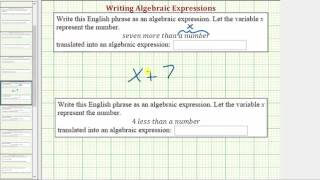Ex: Write a Algebraic Expression in the Form x+c and c-x (less and more)

This video explains how to write a algebraic or variable expression from a given statement. http://mathispower4u.com

From playlist Evaluating and Writing Algebraic Expressions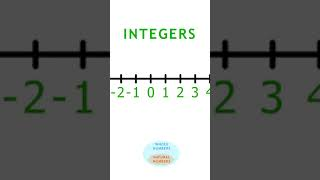Different Types of Numbers on the number line, lesson 1 #shorts

Watch the full playlist: https://www.youtube.com/watch?v=kcxK3_sROZA&list=PL14bv5vXK2WWuODhGbpPQA0GamV5ohOVb&index=1 Natural Numbers (N), (also called positive integers, counting numbers, or natural numbers); They are the numbers {1, 2, 3, 4, 5, …} Whole Numbers (W). This is the set of na

From playlist Celebrities Teach Math: The Number System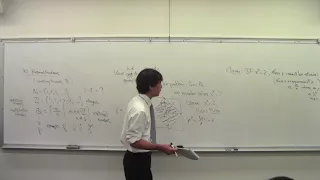Math 101 090417 Introduction to Analysis 02 Rational Numbers

Description of the rational numbers. Proof that the square root of two is not rational. Definition of algebraic number.

From playlist Course 6: Introduction to Analysis (Fall 2017)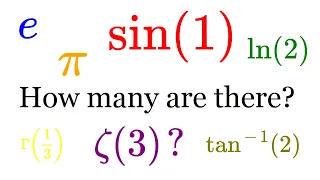Algebraic numbers are countable

Transcendental numbers are uncountable, algebraic numbers are countable. There are two kinds of real numbers: The algebraic numbers (like 1, 3/4, sqrt(2)) and the transcendental numbers (like pi or e). In this video, I show that the algebraic numbers are countable, which means that there

From playlist Real NumbersNumber theory Full Course [A to Z]

Number theory (or arithmetic or higher arithmetic in older usage) is a branch of pure #mathematics devoted primarily to the study of the integers and integer-valued functions. Number theorists study prime numbers as well as the properties of objects made out of integers (for example, ratio

From playlist Number Theory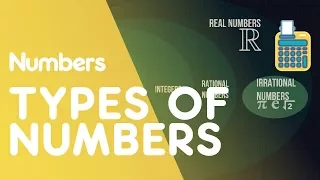Types Of Numbers | Numbers | Maths | FuseSchool

We all know what numbers are 1, 2, 3, 4, 5, …. Including negative numbers -1, -2, -3, -4, -5, ... But did you know that mathematicians classify numbers into different types… into a number system. Let’s start at the top with real numbers. They can be positive… negative… zero… decimals, frac

From playlist MATHS: Numbers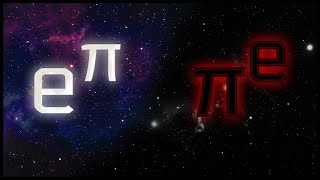Are they irrational? Transcendental? | Epic Math Time

Showing that a number is transcendental can be difficult. While π and e have a deep connection involving exponentiation, other combinations of them, like π + e, are not as well understood. Follow me on Instagram for previews, behind-the-scenes, and more content!: http://instagram.com/epic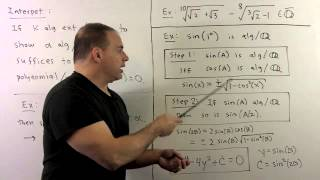FIT2.3.3. Algebraic Extensions

Field Theory: We define an algebraic extension of a field F and show that successive algebraic extensions are also algebraic. This gives a useful criterion for checking algberaic elements. We finish with algebraic closures.

From playlist Abstract Algebra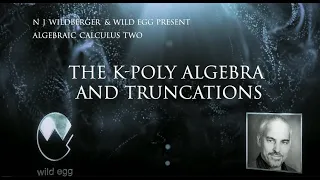The k-Poly Algebra and truncations | Algebraic Calculus Two | Wild Egg Maths

We introduce finite algebraic approximations to the algebra of polynumbers called k-polys, where k is a natural number. The key notion here is that of an algebra: which is a linear or vector space with an additional (associative) multiplication that distributes with the linear structure o

From playlist Algebraic Calculus Two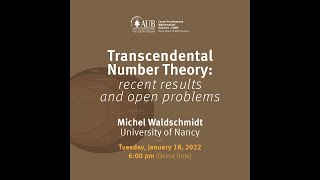"Transcendental Number Theory: Recent Results and Open Problem​s" by Prof. Michel Waldschmidt​

This lecture will be devoted to a survey of transcendental number theory, including some history, the state of the art and some of the main conjectures.

From playlist Number Theory Research Unit at CAMS - AUB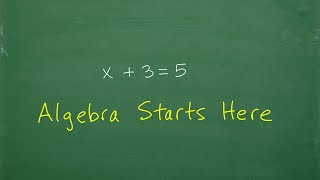Algebra – It All Starts Here…

TabletClass Math: https://tcmathacademy.com/ What is algebra? A introduction to algebra and basic algebra concepts. For more math help to include math lessons, practice problems and math tutorials check out my full math help program at https://tcmathacademy.com/ Math Notes: P

From playlist GED Prep VideosGalois theory: Algebraic closure

This lecture is part of an online graduate course on Galois theory. We define the algebraic closure of a field as a sort of splitting field of all polynomials, and check that it is algebraically closed. We hen give a topological proof that the field C of complex numbers is algebraically

From playlist Galois theory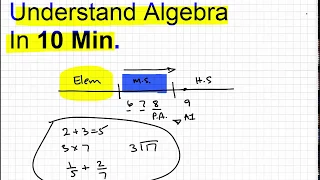Understand Algebra in 10 min

TabletClass Math http://www.tabletclass.com learn the basics of algebra quickly. This video is designed to introduce algebra concepts for all math students and make the topic easy to understand.

From playlist GED Prep Videos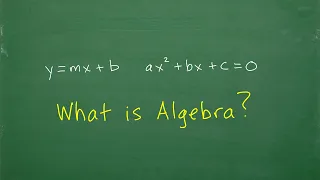What Exactly is Algebra?

TabletClass Math: https://tcmathacademy.com/ What is Algebra? Let's answer this question in a clear and understandable way. For more math help to include math lessons, practice problems and math tutorials check out my full math help program at https://tcmathacademy.com/ Math Note

From playlist GED Prep Videos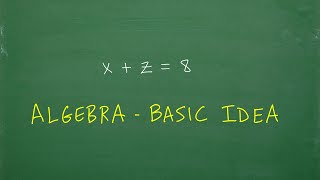Algebra – the most BASIC concepts you need to understand

TabletClass Math: https://tcmathacademy.com/ What is algebra? The basic concepts you learn in algebra. For more math help to include math lessons, practice problems and math tutorials check out my full math help program at https://tcmathacademy.com/ Math Notes: Pre-Algebra No

From playlist GED Prep Videos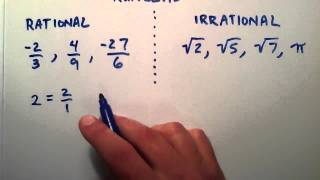What is the Difference Between Rational and Irrational Numbers , Intermediate Algebra , Lesson 12

This tutorial explains the difference between rational and irrational numbers. Join this channel to get access to perks: https://www.youtube.com/channel/UCn2SbZWi4yTkmPUj5wnbfoA/join :)

From playlist Intermediate Algebra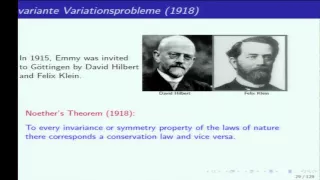Emmy Noether: breathtaking mathematics - Georgia Benkart

Celebrating Emmy Noether Topic: Emmy Noether: breathtaking mathematics Speaker: Georgia Benkart Affiliation: University of Wisconsin-Madison Date: Friday, May 6 By the mid 1920s, Emmy Noether had made fundamental contributions to commutative algebra and to the theory of invariants.

From playlist Celebrating Emmy Noether